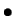>Next: (b) Formula for Rfrequency Up: (a) Calculation of Rsequence Previous: (iii) Determining the Binding

### (iv) Variable Spacing

When a recognition site has two or more parts with various spacings between them, alignment by one part may blur out information in the other part. For example, if the four variants of this site:

 A C G T A C G T A C G T n n n n n n n n G G C C n A C G T A C G T A C G T n n n n n n n G G C C n n A C G T A C G T A C G T n n n n n n G G C C n n n A C G T A C G T A C G T n n n n n G G C Coccurred with equal frequency, then the positions marked by dots would have zero information content, even though these sequences would give a large information content if they were aligned with each other. To handle this one may align each part separately and add the information contents together. However, this leads to an overestimate of the information because the variable spacing is not taken into account. To take it into account, one may calculate how uncertain the spacing is from a tabulation of the frequency of each spacing and subtract this from the total information of the two parts. (This is equivalent to increasing the uncertainty of the site, Hs.) For the example above, Rsequence = 24 (ACGTACGTACGT) + 8 (GGCC) - 2 (spacing) = 30 bits. When this was done for ribosome binding sites, the total information content was not different from that given in Results (unpublished observation).Next: (b) Formula for Rfrequency Up: (a) Calculation of Rsequence Previous: (iii) Determining the Binding
Tom Schneider
2002-10-16